# Multichoice The Muscular System Flashcards

Set Details Share
created 8 years ago by Kieran_Kennedy
14,733 views
Page to share:
Embed this setcancel
COPY
code changes based on your size selection
Size:
X

1

Most muscles cross at least one

a) tendon

b) joint

c) bone

d) ligament

e) body plane

b

2

The attachment of a muscle’s tendon to the stationary bone is called the _____; the attachment of the muscle’s other tendon to the movable bone is called the _____.

a) origin, action

b) insertion, action

c) origin, insertion

d) insertion, origin

e) insertion, action

c

3

A lever is acted on at two different points by two different forces which are called the

a) fulcrum and resistance.

c) lever and resistance.

e) lever and effort.

d

4

Motion will occur in a muscle when the _____ supplied exceeds the _____.

b) resistance, lever

e) lever, effort

a

5

Which of the following is NOT a common arrangement of muscle fascicles?

a) pennate

b) triangular

c) oval

d) parallel

e) fusiform

c

6

This type of fascicle arrangement has the fascicles spread over a broad area and converges at a thick central tendon.

1. a) triangular
2. b) pennate
3. c) circular
4. d) fusiform
5. e) multipennate

a

7

The muscle that serves as the “prime mover” during a movement is called the

a) antagonist.

b) agonist.

c) synergist.

d) asynergist.

e) fixator.

b

8

This type of muscle works by stabilizing the origin of the agonist so that it can act more efficiently.

a) synergist

b) agonist

c) antagonist

d) fixator

e) secondary mover

d

9

Which of the following is NOT a characteristic commonly used to name skeletal muscles?

a) Size

b) Shape

c) Number of origins

d) Sites of origins

e) Thickness of fibers

e

10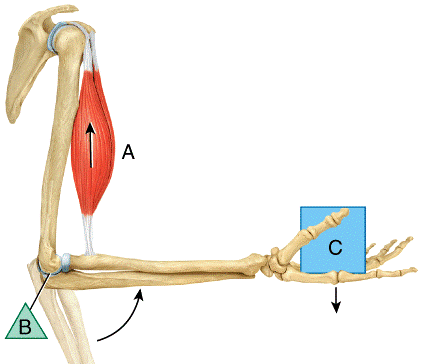In the diagram, where is the fulcrum?

1. a) A
2. b) B
3. c) C
4. d) All of these choices

e) None of these choices

b

11In the diagram, where is the effort?

1. a) A
2. b) B
3. c) C
4. d) All of these choices
5. e) None of these choices

a

12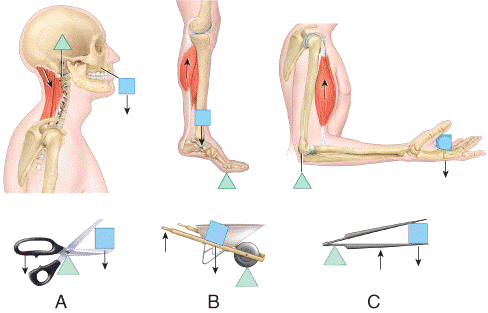Which one represents a second-class lever?

1. a) A
2. b) B
3. c) C
4. d) All of these choices
5. e) None of these choices

b

13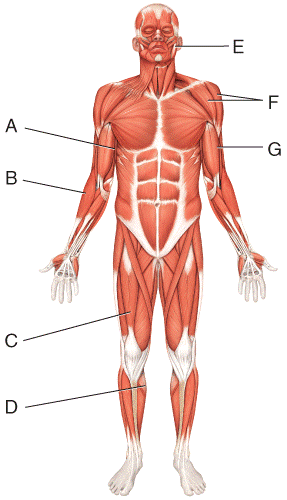Where is the rectus femoris?

1. a) A
2. b) B
3. c) C
4. d) D
5. e) I

c

14Where is the deltoid?

1. a) C
2. b) D
3. c) F
4. d) G
5. e) I

c

15) Where is the masseter?

1. a) A
2. b) B
3. c) G
4. d) I
5. e) E

e

16Where is the gastrocnemius?

1. a) C
2. b) D
3. c) G
4. d) H
5. e) I

b

17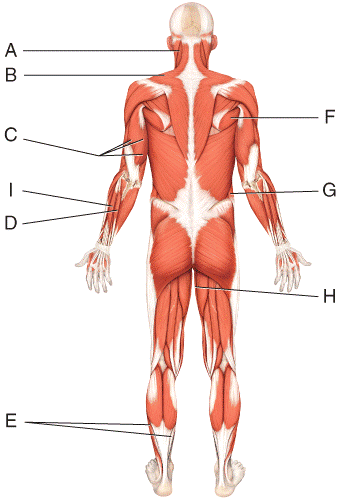Where is the soleus?

1. a) A
2. b) C
3. c) E
4. d) I
5. e) H

c

18Where is the external oblique?

1. a) D
2. b) G
3. c) H
4. d) I
5. e) E

b

19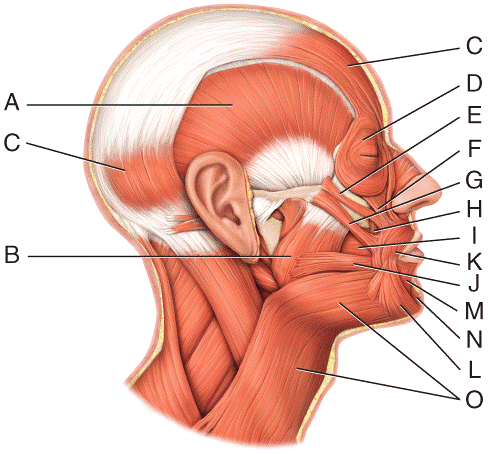Where is the masseter?

1. a) A
2. b) B
3. c) F
4. d) G
5. e) H

b

20Where is the orbicularis oculi?

1. a) D
2. b) E
3. c) F
4. d) G
5. e) H

a

21Where is the buccinator?

1. a) F
2. b) G
3. c) I
4. d) J
5. e) K

c

22Where is the mentalis?

1. a) J
2. b) K
3. c) L
4. d) M
5. e) N

e

23Where is the depressor anguli oris?

1. a) K
2. b) L
3. c) M
4. d) N
5. e) None of these choices

b

24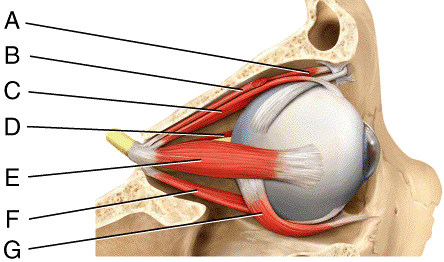Where is the levator palpebrae superioris?

1. a) B
2. b) D
3. c) E
4. d) F
5. e) G

a

25Where is the lateral rectus?

1. a) C
2. b) D
3. c) E
4. d) F
5. e) G

c

26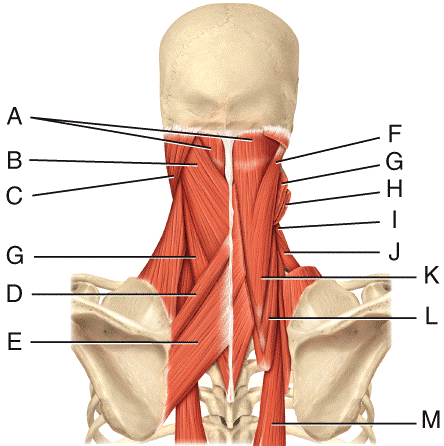Where is the splenius capitis?

1. a) A
2. b) B
3. c) C
4. d) K
5. e) L

b

27Where is the levator scapulae?

1. a) H
2. b) F
3. c) I
4. d) L
5. e) A

a

28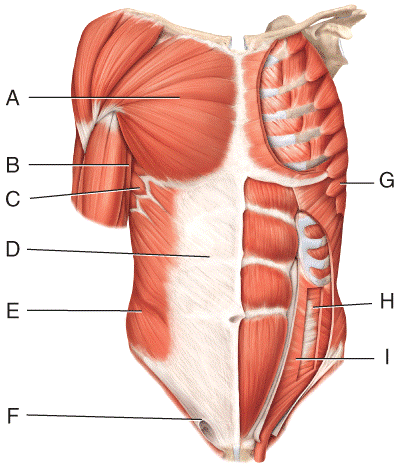Where is the serratus anterior?

1. a) A
2. b) B
3. c) C
4. d) D
5. e) G

c

29Where is the external oblique?

1. a) D
2. b) E
3. c) F
4. d) H
5. e) I

b

30Where is the transverse abdominus?

1. a) D
2. b) E
3. c) F
4. d) H
5. e) I

d

31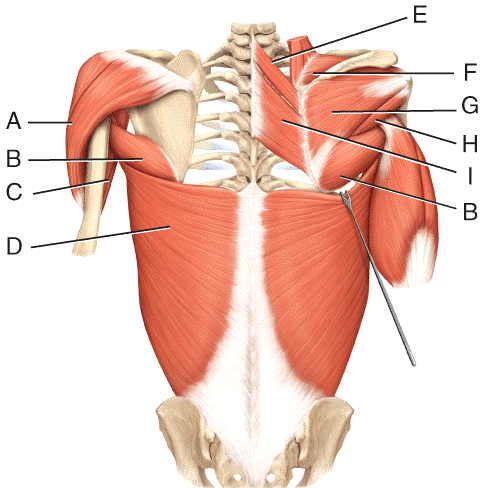Where is the infraspinatus?

1. a) E
2. b) F
3. c) G
4. d) H
5. e) I

c

32Where is the rhomboid major?

1. a) E
2. b) F
3. c) G
4. d) H
5. e) I

e

33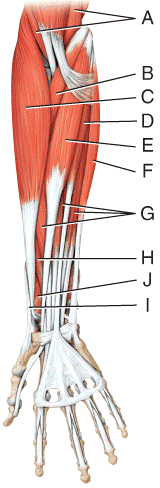1. a) A
2. b) B
3. c) C
4. d) D
5. e) E

c

34Where is the flexor carpi radialis?

1. a) D
2. b) E
3. c) F
4. d) G
5. e) H

b

35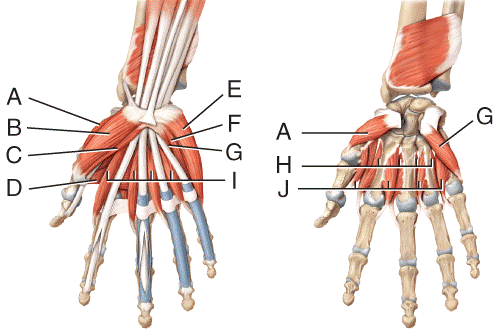Where is the abductor digiti minimi?

1. a) B
2. b) C
3. c) E
4. d) F
5. e) G

c

36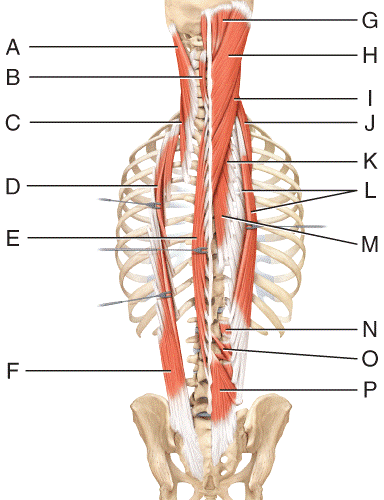Where is the splenius capitis?

1. a) A
2. b) B
3. c) C
4. d) H
5. e) I

e

37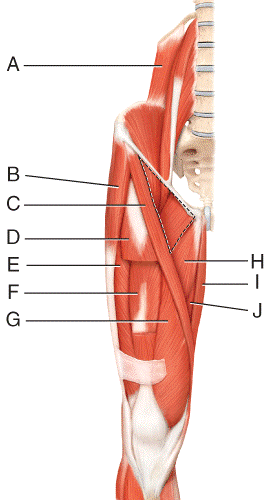Where is the iliacus?

1. a) A
2. b) B
3. c) C
4. d) H
5. e) None of these choices

a

38Where is the sartorius?

1. a) A
2. b) B
3. c) C
4. d) D

e) None of these choices

c

39Where is the vastus intermedius?

1. a) D
2. b) E
3. c) F
4. d) G

e) H

c

401. a) H
2. b) I
3. c) J
4. d) K
5. e) L

a

41Where is the gracilis?

1. a) H
2. b) I
3. c) J
4. d) K
5. e) L

b

42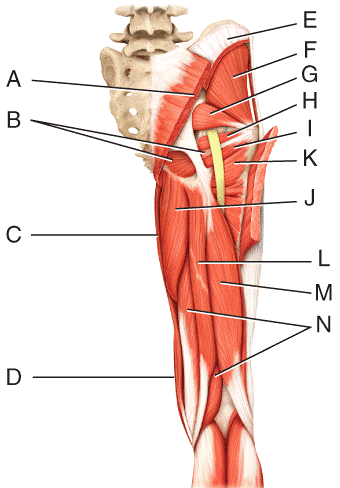) Which three muscles make up the hamstring?

1. a) L, M, N
2. b) E, F, G
3. c) H, I, J
4. d) I, J, K
5. e) A, B, C

a

43Where is the semitendinosus?

1. a) J
2. b) K
3. c) L
4. d) M
5. e) N

c

44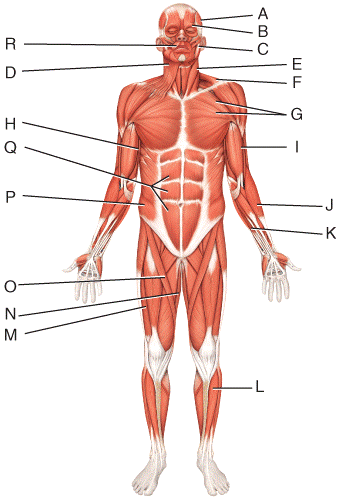What is the origin of the muscle labeled B?

1. a) upper lip
2. b) mandible
3. c) medial wall of orbit
4. d) skin at the corner of the mouth
5. e) skin of the eyebrow

c

45What is the origin of the muscle labeled D?

1. a) fascia over parotid gland
2. b) fascia over deltoid and pectoralis muscles
3. c) skin of the lower lip
4. d) epicranial aponeurosis
5. e) mandible

b

46What is the action of the muscle labeled C?

1. a) laterally flex and rotate head
3. c) depresses tongue
4. d) protracts mandible (as in opening the mouth)
5. e) elevates the mandible (as in closing the mouth)

e

47What is the insertion of the muscle labeled E?

1. a) mastoid process of temporal bone
2. b) sternum and clavicle
3. c) occipital bone
4. d) clavicle and spine of the scapula
5. e) coracoid process of the scapula

a

48What is the action of the muscle labeled G?

1. a) adducts and medially rotates arm at shoulder joint
2. b) abducting arm at shoulder joint
3. c) laterally rotates arm at shoulder joint
4. d) laterally rotates and extends arm at shoulder joint
5. e) extends forearm at elbow joint

a

49What is the action of the muscle labeled I?

1. a) extends the forearm at the elbow joint
2. b) flexes the forearm at the elbow joint
3. c) pronates the forearm at the radioulnar joints
4. d) abduct arm at shoulder joint
5. e) rotates arm at shoulder joint

b

50What is the insertion of the muscle labeled Q?

1. a) iliac crest and linea alba
2. b) cartilage of the fifth to seventh rib and xiphoid process
3. c) ribs 5-12
4. d) central tendon
5. e) costal cartilages and adjacent portion of the inferior 6 ribs

b

51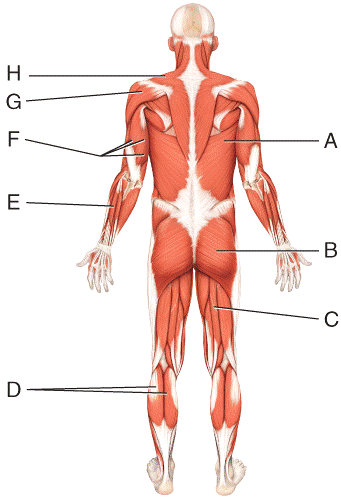What is the insertion of the muscle labeled F?

1. a) ulnar tuberosity and coronoid process of ulna
3. c) olecranon of ulna
4. d) lateral border of distal end of humerus
5. e) lateral epicondyle of humerus

c

52What is the insertion of the muscle labeled G?

1. a) greater tubercle of humerus
2. b) inferior angle of scapula
3. c) spine of the scapula
4. d) acromial extremity of the clavicle
5. e) deltoid tuberosity of humerus

e

53What is the insertion of the muscle labeled D?

1. a) lateral and medial condyles of femur and capsule of knee
3. c) calcaneus by way of Achilles tendon
4. d) anterior surface of fibula and interosseous membrane
5. e) distal phalanx of great toe

c

54

If one is stabbed on the lateral side of the abdomen, what abdominal muscles, in order from superficial to deep, will the knife go through?

1. a) rectus abdominis, external oblique, internal oblique
2. b) external oblique, internal oblique, transversus abdominis
3. c) transversus abdominis, rectus abdominis, external oblique
4. d) external oblique, transversus abdominis, rectus abdominis
5. e) internal oblique, transversus abdominis, external oblique

b

55

Protrusion of an organ through a structure that normally contains it is referred to as a

a) hernia.

b) goiter.

c) strain.

d) sprain.

e) hydrocele.

a

56

A condition where the two eyeballs are not properly aligned is referred to as

1. a) inguinal hernia.
2. b) strabismus.
3. c) presbyopia.
4. d) myopia.
5. e) hypermetropia.

b

57

A muscle that has three origins is called a

1. a) biceps.
2. b) triceps.
4. d) deltoid.
5. e) trapezius.

b

58

A muscle that raises or elevates a body part is called a

1. a) pronator.
2. b) tensor.
3. c) flexor.
4. d) depressor.
5. e) levator.

b

59

A muscle that raises or elevates a body part is called a

1. a) pronator.
2. b) tensor.
3. c) flexor.
4. d) depressor.
5. e) levator.

b

60

) A muscle that decreases the size of an opening is a

a) rotator.

b) tensor.

c) pronator.

d) sphincter.

e) depressor.

d

61

Contraction of this muscle presses the cheeks against the teeth and lips as would be observed in whistling, blowing or sucking.

a) buccinator

b) zygomaticus

c) frontalis

d) epicranius

e) occipitalis

a

62

All of the following are muscles that move the eyeball EXCEPT

a) superior rectus.

b) lateral rectus.

c) inferior oblique.

d) internal oblique.

e) superior oblique.

d

63

Which of the following is a muscle whose insertion is found on the clavicle and acromion process of the scapula within the pectoral girdle?

1. a) trapezius
2. b) pectoralis major
3. c) latissimus dorsi
4. d) gracilus
5. e) sartorius

a

64

All of the following are muscles of the lower limb EXCEPT the

a) deltoid.

e) vastus medialis.

a

65

Based on its name, which of the following best describes characteristics of the biceps femoris muscle?

a) has two insertions and found in lower leg

b) has two bellies and found in the upper leg

c) has two origins and found in the upper leg

d) has two origins and found in the upper arm

e) has two insertions and found in the upper leg

c

66

Based on its name, which of the following best describes characteristics of the biceps femoris muscle?

a) has two insertions and found in lower leg

b) has two bellies and found in the upper leg

c) has two origins and found in the upper leg

d) has two origins and found in the upper arm

e) has two insertions and found in the upper leg

c

67

Which of the following muscles is NOT part of the quadriceps femoris muscle group?

1. a) rectus femoris
2. b) biceps femoris
3. c) vastus medialis
4. d) vastus lateralis
5. e) vastus intermedius

b

68

This muscle, which is found in the neck, functions to elevate the hyoid bone and help press the tongue against the roof of the mouth during swallowing.

a) mylohyoid

b) sternothryroid

c) sternocleidomastoid

d) digastric

e) masseter

a